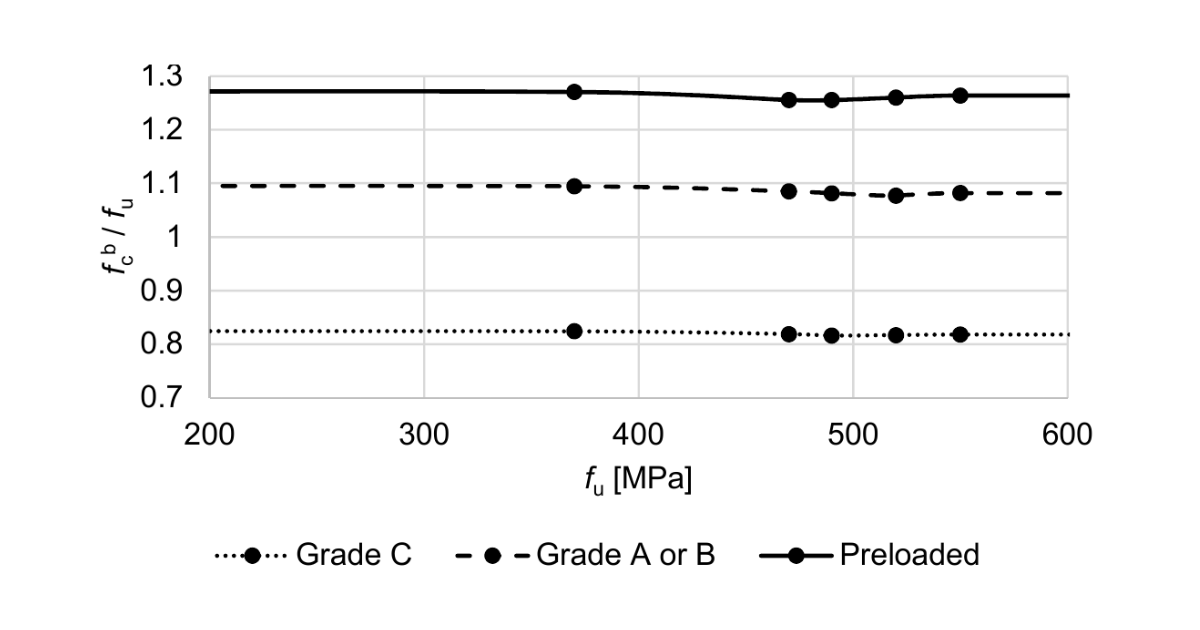### Choose language# Code-check of bolts and preloaded bolts according to Chinese standard

$$BoltsBolts are checked according to GB 50017, Cl. 11.4. The tensile and shear force in each bolt is determined by finite element analysis. Prying forces are determined by finite element analysis and taken into account. Each shear plane is checked individually. The plate in the bearing is checked against the sum of shear forces at nearby planes.Design tensile and shear strengths of a bolt; fub[MPa] – ultimate strength of a bolt; derived from Table 4.4.6 $$f_{ub}$$ [MPa]$$f_t^b$$$$f_v^b$$ $$f_{ub} \le 400$$$$0.425 \cdot f_{ub}$$$$0.35 \cdot f_{ub}$$ \(400